Courses

# Test: Physics- 11

## 45 Questions MCQ Test NEET Mock Test Series | Test: Physics- 11

Description
This mock test of Test: Physics- 11 for NEET helps you for every NEET entrance exam. This contains 45 Multiple Choice Questions for NEET Test: Physics- 11 (mcq) to study with solutions a complete question bank. The solved questions answers in this Test: Physics- 11 quiz give you a good mix of easy questions and tough questions. NEET students definitely take this Test: Physics- 11 exercise for a better result in the exam. You can find other Test: Physics- 11 extra questions, long questions & short questions for NEET on EduRev as well by searching above.
QUESTION: 1

Solution:
QUESTION: 2

Solution:
QUESTION: 3

### A person brings a mass of 1 kg from infinity to a point A. Initially the mass was at rest but it moves at a speed of 2m/s as it reaches A. The work done by the person on the mass is –3J. The potential at A is

Solution:
QUESTION: 4
The time period of an earth-satellite in circular orbit is independent of
Solution:
QUESTION: 5

Figure shown the elliptical path of a planet about the sun. The two shaded parts have equal area. If tand tbe the time taken by the planet to go from a to b and from c to d respectively,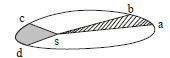Solution:
QUESTION: 6

A particle is kept at rest at a distance R(earth’s radius) above the earth’s surface. The minimum speed with which it should be projected so that it does not return is

Solution: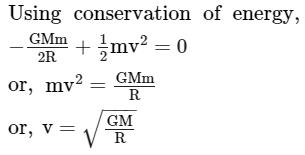QUESTION: 7
A uniform spherical shell gradually shrinks maintaining its shape. The gravitational potential at the center
Solution:
QUESTION: 8

Two satellites A and B move round the earth in the same orbit. The mass of B is twice the mass of A

Solution:
QUESTION: 9
The motion of a particle is given by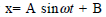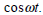The motion of the particle is
Solution:
QUESTION: 10
A particle executes simple harmonic motion with a frequency v. The frequency with which the kinetic
energy oscillates is
Solution:
QUESTION: 11

A particle executes simple harmonic motion under the restoring force provided by a spring. The time period is T. If the spring is divided in two equal parts and one part is used to continue the simple harmonic motion , the time period will

Solution:
QUESTION: 12

The free end of a simple pendulum is attached to the ceiling of a box. The box is taken to a height and the point, the box is released to fall freely. As seen from the box during this period, the bob will

Solution:
QUESTION: 13
A particale moves in a circular path with a uniform speed. Its motion is
Solution:
QUESTION: 14
Which of the following quantities are always zero in a simple harmonic motion ?
Solution:
QUESTION: 15

A particle moves on the x-axis according to the equation x = x0 sin2ωt. The motion is simple harmonic

Solution:
QUESTION: 16

A sine wave is travelling in a medium. A particular particle has zero displacement at a certain instant. The particle closest to it having zero displacement is at a distance

Solution:
QUESTION: 17

Both the strings, shown in figure are made of same material and have same cross-section. The pulleys are light. The wave speed of a transverse wave in the string AB is vand in CD it is v2. Then v1/v2 is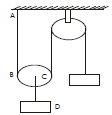Solution:
QUESTION: 18

A wave pulse, travelling on a two-piece string, gets partially reflected and Partially transmitted at the Junction. The reflected wave is inverted in shape as compared to the incident one. If the incident wave has wavelength λ and the transmitted wave λ

Solution:
QUESTION: 19

Two waves represented by y = a sin (ωt-kx) and y = a cos(ωt-kx)  are superposed. The resultant wave will have an amplitude

Solution:
QUESTION: 20

Consider two waves passing through the same string.Principle of superposition for displacement says that the net displacement of a particle on the string is sum of the displacements proudced by the two waves individually. Suppose we state similar principles for the net velocity of the particle and the kinetic energy of the particle. Such a principle will be valid for

Solution:
QUESTION: 21

Two sine waves travel in the same direction in a medium.The amplitude of each wave is A and the phase difference between the two waves is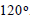The resultant amplitude will be

Solution:
QUESTION: 22

A sonometer wire of length l vibrates in fundamental mode when excited by a tuning fork of frequency 416Hz. If the length is doubled keeping other things same,the string will

Solution:
QUESTION: 23
Longitudinal waves cannot
Solution:
QUESTION: 24

Consider the following statements about sound passing through a gas
A. The pressure of the gas at a point oscillates in time
B. The position of a small layer of the gas oscillates in time

Solution:
QUESTION: 25

A tuning fork sends sound waves in air. If the temperature of the air increases, which of the following parameters will change ?

Solution:
QUESTION: 26

The speed of sound in a medium depends on

Solution:
QUESTION: 27
An organ pipe, open at both ends, contains
Solution:
QUESTION: 28
A cylindrical tube, open at both ends, has a fundamental frequency v. The tube is dipped vertically in water so that half of its length is inside the water. The new fundamental frequency is
Solution:
QUESTION: 29

A tuning fork of frequency 512 Hz is vibrated with a sonometer wire and 6 beats per second are heard. The beat frequency reduces if the tension in the string is slightly increased. The original frequency of vibration of the string is

Solution:

f1 = 512Hz
f2 = frequency of sonometer wire.
Now, 512 -f2 = 6
By increasing the tension in sonometer wire fwill increase.
In first case, beat frequency will decreae.
∴ 512 - f2 = 6
or f2 = 506 Hz

QUESTION: 30

A small sources of sound moves on a circle as shown in figure and an observer is sitting at O.Let  v1,v2,vbe the frequencies heard when the source is at A,B and C respectively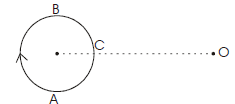Solution:
QUESTION: 31
When you speak to your friend, which of the following parameters have a unique value in the sound produced?
Solution:
QUESTION: 32

The fundamental frequency of a vibrating organ pipe is 200 Hz

Solution:
QUESTION: 33

A simple pendulum with a brass bob has a time period T. The bob is now immersed in a non-viscous liquid and oscillated. If the density of the liquid is 1/8 that of brass, the time period of the same pendulum will be

Solution: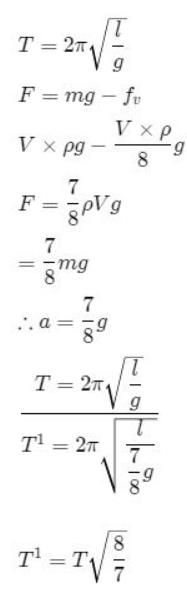QUESTION: 34
In the figure, the block of mass m, attached to the spring of stiffness k is in contact with the completely elastic wall, and the compression in the spring is e.The spring is compressed further by e by displacing the block towards left and is, then released. If the collision between the block and the wall is completely elastic, then the time period of oscillations of the block will be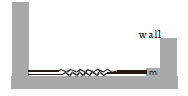Solution:
QUESTION: 35

Two sounding bodies producing progressive waves given by y1 = 4sin(400πt) and y2 = 3sin(404πt) where t is in seconds, are situated near the ears of a person. The person will hear

Solution:
QUESTION: 36

A closed organ pipe of length L and an open organ contain gases of densities ρ1 and ρrespectively.The compressibility of gases are equal in both the pipes. Both the pipes are vibrating in their first overtone with same frequency. The length of the organ pipe is

Solution:
QUESTION: 37

A wire of 9.8 x 10-3kgm-1 passes over a frinctionless light pulley fixed on the top of frictionless inclined plane which makes an angle of 30with the horizontal. Masses m and M are tied at the two ends of wire such that m rests on the plane and M hangs freely vertically downwards. The entire system is in equilibrium and a transverse wave propagates along the wire with a velocity of 100 ms-1.Choose the correct option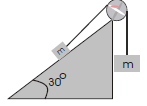Solution:
QUESTION: 38
Direction : Read the following question and choose
A. If both Assertion and Reason are correct and reason is the correct explanation of assertion
B. If both Assertion and Reason are true, but Reason is not correct explanation of the Assertion
C. If Assertion is true, but the Reason is false
D. If Assertion is false, but the Reason is true
E. If both assertion and reason are false
Assertion : Compression and rarefaction involve changes in density and pressure
Reason : When particles are compressed, density of medium increases and when they are rerefied, density of medium decrease
Solution:
QUESTION: 39
Direction : Read the following question and choose
A. If both Assertion and Reason are correct and reason is the correct explanation of assertion
B. If both Assertion and Reason are true, but Reason is not correct explanation of the Assertion
C. If Assertion is true, but the Reason is false
D. If Assertion is false, but the Reason is true
E. If both assertion and reason are false
Assertion : On a rainy day sound travel slower than on a dry day
Reason : When moisture is present in air the density of air increases
Solution:
QUESTION: 40
Direction : Read the following question and choose
A. If both Assertion and Reason are correct and reason is the correct explanation of assertion
B. If both Assertion and Reason are true, but Reason is not correct explanation of the Assertion
C. If Assertion is true, but the Reason is false
D. If Assertion is false, but the Reason is true
E. If both assertion and reason are false
Assertion : An open organ pipe of certain length have the same fundamental frequency as closed organ pipe of half the length
Reason : In the case of open oran pipe, at both the ends antinodes are formed, while in the closed organ pipe at one end antinode and at the other node is formed
Solution:
QUESTION: 41
Direction : Read the following question and choose
A. If both Assertion and Reason are correct and reason is the correct explanation of assertion
B. If both Assertion and Reason are true, but Reason is not correct explanation of the Assertion
C. If Assertion is true, but the Reason is false
D. If Assertion is false, but the Reason is true
E. If both assertion and reason are false
Assertion : We can recognise our friends by listening their voices
Reason : The quality of sound produced by different persons are different
Solution:
QUESTION: 42
Direction : Read the following question and choose
A. If both Assertion and Reason are correct and reason is the correct explanation of assertion
B. If both Assertion and Reason are true, but Reason is not correct explanation of the Assertion
C. If Assertion is true, but the Reason is false
D. If Assertion is false, but the Reason is true
E. If both assertion and reason are false
Assertion : In simple harmonic total mechanical energy can be negative also
Reason : Potential energy is always negative and if it is greater than kinetic energy total mechanical energy will be negative
Solution:
QUESTION: 43
Direction : Read the following question and choose
A. If both Assertion and Reason are correct and reason is the correct explanation of assertion
B. If both Assertion and Reason are true, but Reason is not correct explanation of the Assertion
C. If Assertion is true, but the Reason is false
D. If Assertion is false, but the Reason is true
E. If both assertion and reason are false
Assertion : In SHM let x be the maximum speed, y the frequency of oscillation and z the maximum acceleration then xy /z is a constant quanitity
Reason : The is because xy /z becomes a dimensionless quantiy
Solution:
QUESTION: 44
Direction : Read the following question and choose
A. If both Assertion and Reason are correct and reason is the correct explanation of assertion
B. If both Assertion and Reason are true, but Reason is not correct explanation of the Assertion
C. If Assertion is true, but the Reason is false
D. If Assertion is false, but the Reason is true
E. If both assertion and reason are false
Assertion : At height h from ground and at depth h below ground, where h is approximately equal to 0.62 R, the value of g acceleration due to gravity is same
Reason : Value of g decreases both sides, in going up and down
Solution:
QUESTION: 45
Direction : Read the following question and choose
A. If both Assertion and Reason are correct and reason is the correct explanation of assertion
B. If both Assertion and Reason are true, but Reason is not correct explanation of the Assertion
C. If Assertion is true, but the Reason is false
D. If Assertion is false, but the Reason is true
E. If both assertion and reason are false
Assertion : For a system of masses, at some finite distance gravitational field can be zero but gravitational potential cannot
Reason : Gravitational field is a vector quantity, while potential a scalar one
Solution: